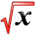# Some Math TipsThis article is part of the MathHelp Tutoring Wiki
Question: How to factor $x^{2}+bx\pm c$without quadratic formulas (b,c are integers)
Answer: A trick to factor such equations is that we find two factors of c such they add up to b (if + sign) or subtract to b(if - sign) e.g factor $x^{2}+5x-6$. Now 6 has two factors: 6 and 1 which subtract to 5. so using this trick, we can write $x^{2}+6x-x-6$, which gives $(x+6)(x-1)$